# Interactive Mini-Lessons and Simulations

Interactive lessons that provide ways for students to manipulate data and use interactive tools to gain a deeper understanding of complex topics.
435 affiliated resources
View
Selected filters: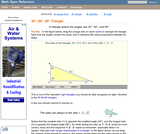Conditions of Use:
Read the Fine Print
Rating

An interactive applet and associated web page that demonstrate the properties of a 30-60-90 triangle. The applet shows a right triangle that can be resized by dragging any vertex. As it is dragged, the remaining vertices change so that the triangle's angles remain 30 degrees, 60 degrees and 90 degrees The text on the page points out that the sides of a 30-60-90 triangle are always in the ratio of 1 : 2 : root 3 Applet can be enlarged to full screen size for use with a classroom projector. This resource is a component of the Math Open Reference Interactive Geometry textbook project at http://www.mathopenref.com.

Subject:
Geometry
Material Type:
Reading
Simulation
Provider:
Math Open Reference
Author:
John Page
Date Added:
02/16/2011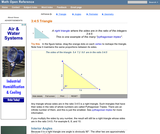Conditions of Use:
Read the Fine Print
Rating

An interactive applet and associated web page that demonstrate the properties of a 3:4:5 triangle - one of the Pythagorean triples. The applet shows a right triangle that can be resized by dragging any vertex. As it is dragged, the remaining vertices change so that the triangle's side remain in the ration 3:4:5. The text on the page has an example of how the triangle can be used to measure a right angle on even large objects. Applet can be enlarged to full screen size for use with a classroom projector. This resource is a component of the Math Open Reference Interactive Geometry textbook project at http://www.mathopenref.com.

Subject:
Geometry
Material Type:
Reading
Simulation
Provider:
Math Open Reference
Author:
John Page
Date Added:
02/16/2011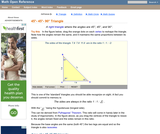Conditions of Use:
Read the Fine Print
Rating

An interactive applet and associated web page that demonstrate the properties of a 45-45-90 isosceles right triangle. The applet shows a right triangle that can be resized by dragging any vertex. As it is dragged, the remaining vertices change so that the triangle's angles remain 45 degrees, 45 degrees and 90 degrees The text on the page points out that the sides of a 45-45-90 triangle are always in the ratio of 1 : 2 : root 2 Applet can be enlarged to full screen size for use with a classroom projector. This resource is a component of the Math Open Reference Interactive Geometry textbook project at http://www.mathopenref.com.

Subject:
Geometry
Material Type:
Reading
Simulation
Provider:
Math Open Reference
Author:
John Page
Date Added:
02/16/2011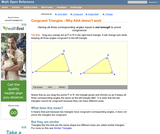Conditions of Use:
Read the Fine Print
Rating

An interactive applet and associated web page that shows that angle-angle-angle (AAA) is not enough to prove congruence. The applet shows two triangles, one of which can be dragged to resize it, showing that although they have the same angles they are not the same size and thus not congruent. The web page describes all this and has links to other related pages. Applet can be enlarged to full screen size for use with a classroom projector. This resource is a component of the Math Open Reference Interactive Geometry textbook project at http://www.mathopenref.com.

Subject:
Geometry
Material Type:
Reading
Simulation
Provider:
Math Open Reference
Author:
John Page
Date Added:
02/16/2011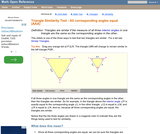Conditions of Use:
Read the Fine Print
Rating

An interactive applet and associated web page showing how the AAA similarity test works. Two similar triangles are shown that can be resized by dragging. The other triangle adjusts to remain similar and the angle-angle-angle elements are highlighted to show how they are involved in this test of similarity. (all three interior angles congruent). The web page describes all this and has links to other related pages. Applet can be enlarged to full screen size for use with a classroom projector. This resource is a component of the Math Open Reference interactive geometry reference book project at http://www.mathopenref.com.

Subject:
Geometry
Material Type:
Reading
Simulation
Provider:
Math Open Reference
Author:
John Page
Date Added:
02/16/2011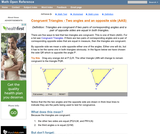Conditions of Use:
Read the Fine Print
Rating

An interactive applet and associated web page that shows how triangles that have two angles and a non-included side the same must be congruent. The applet shows two triangles, one of which can be reshaped by dragging any vertex. The other changes to remain congruent to it and the two angles and non-included side are outlined in bold to show they are the same measure and are the elements being used to prove congruence. The web page describes all this and has links to other related pages. Applet can be enlarged to full screen size for use with a classroom projector. This resource is a component of the Math Open Reference Interactive Geometry textbook project at http://www.mathopenref.com.

Subject:
Geometry
Material Type:
Reading
Simulation
Provider:
Math Open Reference
Author:
John Page
Date Added:
02/16/2011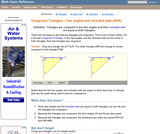Conditions of Use:
Read the Fine Print
Rating

An interactive applet and associated web page that shows how triangles that have two angles and their included side the same must be congruent. The applet shows two triangles, one of which can be reshaped by dragging any vertex. The other changes to remain congruent to it and the two angles and the included side are outlined in bold to show they are the same measure and are the elements being used to prove congruence. The web page describes all this and has links to other related pages. Applet can be enlarged to full screen size for use with a classroom projector. This resource is a component of the Math Open Reference Interactive Geometry textbook project at http://www.mathopenref.com.

Subject:
Geometry
Material Type:
Reading
Simulation
Provider:
Math Open Reference
Author:
John Page
Date Added:
02/16/2011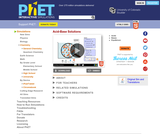Conditions of Use:
No Strings Attached
Rating

How do strong and weak acids differ? Use lab tools on your computer to find out! Dip the paper or the probe into solution to measure the pH, or put in the electrodes to measure the conductivity. Then see how concentration and strength affect pH. Can a weak acid solution have the same pH as a strong acid solution?

Subject:
Chemistry
Material Type:
Simulation
Provider:
University of Colorado Boulder
Provider Set:
PhET Interactive Simulations
Author:
Chris Malley
Kathy Perkins
Kelly Lancaster
Patricia Loeblein
Robert Parson
Date Added:
09/01/2010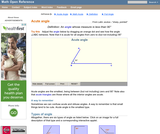Conditions of Use:
Read the Fine Print
Rating

An interactive applet and associated web page that demonstrate acute angles (those less than 90 deg). The applet presents an angle (initially acute) that the user can adjust by dragging the end points of the line segments forming the angle. As it changes it shows the angle measure and a message that indicate which type of angle it is. There a software 'detents' that make it easy capture exact angles such as 90 degrees and 180 degrees The message and angle measures can be turned off to facilitate classroom discussion. The text on the page has links to other pages defining each angle type in depth. Applet can be enlarged to full screen size for use with a classroom projector. This resource is a component of the Math Open Reference Interactive Geometry textbook project at http://www.mathopenref.com.

Subject:
Geometry
Material Type:
Reading
Simulation
Provider:
Math Open Reference
Author:
John Page
Date Added:
02/16/2011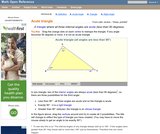Conditions of Use:
Read the Fine Print
Rating

An interactive applet and associated web page that demonstrate the three types of triangle: acute, obtuse and right. The applet shows a triangle that is initially acute (all angles less then 90 degrees) which the user can reshape by dragging any vertex. There is a message changes in real time while the triangle is being dragged that tells if the triangle is an acute, right or obtuse triangle and gives the reason why. By experimenting with the triangle student can develop an intuitive sense of the difference between these three classes of triangle. Applet can be enlarged to full screen size for use with a classroom projector. This resource is a component of the Math Open Reference Interactive Geometry textbook project at http://www.mathopenref.com.

Subject:
Geometry
Material Type:
Reading
Simulation
Provider:
Math Open Reference
Author:
John Page
Date Added:
02/16/2011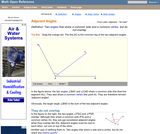Conditions of Use:
Read the Fine Print
Rating

An interactive applet and associated web page that show the concept of adjacent angles (two angles that share a common leg). The applet shows three line segments with a common endpoint. The user can move the center one and see that the angles on both sides (the adjacent angles) of it are affected. Applet can be enlarged to full screen size for use with a classroom projector. After use in the classroom, students can access it again from any web browser at home or in the library with no login required. This resource is a component of the Math Open Reference Interactive Geometry textbook project at http://www.mathopenref.com.

Subject:
Geometry
Material Type:
Reading
Simulation
Provider:
Math Open Reference
Author:
John Page
Date Added:
02/16/2011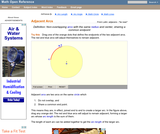Conditions of Use:
Read the Fine Print
Rating

An interactive applet and associated web page that demonstrate the the concept of adjacent arcs. The user can 3 drag points around a circle that define the endpoints of two adjacent arcs. Web page has the properties of adjacent arcs. Applet can be enlarged to full screen size for use with a classroom projector. This resource is a component of the Math Open Reference Interactive Geometry textbook project at http://www.mathopenref.com.

Subject:
Geometry
Material Type:
Reading
Simulation
Provider:
Math Open Reference
Author:
John Page
Date Added:
02/16/2011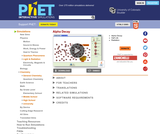Conditions of Use:
No Strings Attached
Rating

Watch alpha particles escape from a polonium nucleus, causing radioactive alpha decay. See how random decay times relate to the half life.

Subject:
Physical Science
Material Type:
Simulation
Provider:
University of Colorado Boulder
Provider Set:
PhET Interactive Simulations
Author:
Carl Wieman
Danielle Harlow
John Blanco
Kathy Perkins
Noah Podolefsky
Ron LeMaster
Sam McKagan
Wendy Adams
Date Added:
07/21/2011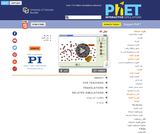Conditions of Use:
Read the Fine Print
Rating

Watch alpha particles escape from a polonium nucleus, causing radioactive alpha decay. See how random decay times relate to the half life.

Subject:
Physical Science
Material Type:
Simulation
Provider:
University of Colorado Boulder
Provider Set:
PhET Interactive Simulations
Author:
Carl Wieman
Danielle Harlow
John Blanco
Kathy Perkins
Noah Podolefsky
Ron LeMaster
Sam McKagan
Wendy Adams
Date Added:
09/02/2012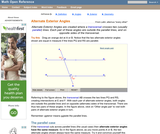Conditions of Use:
Read the Fine Print
Rating

An interactive applet and associated web page that demonstrate the alternate exterior angles that are formed where a transversal crosses two lines. The applets shows the two possible pairs of angles alternating when in animation mode. By dragging the three lines, it can be seen that the angles are congruent only when the lines are parallel. When not in animated mode, there is a button that alternates the two pairs of angles. The text on the page discusses the properties of the angle pairs both in the parallel and non-parallel cases. Applet can be enlarged to full screen size for use with a classroom projector. This resource is a component of the Math Open Reference Interactive Geometry textbook project at http://www.mathopenref.com.

Subject:
Geometry
Material Type:
Reading
Simulation
Provider:
Math Open Reference
Author:
John Page
Date Added:
02/16/2011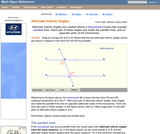Conditions of Use:
Read the Fine Print
Rating

An interactive applet and associated web page that demonstrate the alternate interior angles that are formed where a transversal crosses two lines. The applets shows the two possible pairs of angles alternating when in animation mode. By dragging the three lines, it can be seen that the angles are congruent only when the lines are parallel. When not in animated mode, there is a button that alternates the two pairs of angles. The text on the page discusses the properties of the angle pairs both in the parallel and non-parallel cases. Applet can be enlarged to full screen size for use with a classroom projector. This resource is a component of the Math Open Reference Interactive Geometry textbook project at http://www.mathopenref.com.

Subject:
Geometry
Material Type:
Reading
Simulation
Provider:
Math Open Reference
Author:
John Page
Date Added:
02/16/2011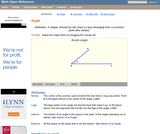Conditions of Use:
Read the Fine Print
Rating

An interactive applet and associated web page that introduce the concept of an angle. An angle made from two line segments is shown that the user can adjust by dragging the end points of the segments. In real time, as the angles is changed by the user, the angle measure in degrees is shown and a message telling what type of angle it currently is: acute, right, obtuse, reflex or straight. Applet can be enlarged to full screen size for use with a classroom projector. This resource is a component of the Math Open Reference Interactive Geometry textbook project at http://www.mathopenref.com.

Subject:
Geometry
Material Type:
Reading
Simulation
Provider:
Math Open Reference
Author:
John Page
Date Added:
02/16/2011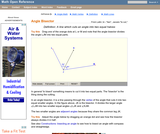Conditions of Use:
Read the Fine Print
Rating

An interactive applet and associated web page that demonstrate the bisector of an angle. An angle is shown using two line segments that can be dragged to change the angle measure. The angle is bisected by a line which moves while dragging to always divide the angle into two equal angles. The angle measures can be turned off for class discussions. Applet can be enlarged to full screen size for use with a classroom projector. This resource is a component of the Math Open Reference Interactive Geometry textbook project at http://www.mathopenref.com.

Subject:
Geometry
Material Type:
Reading
Simulation
Provider:
Math Open Reference
Author:
John Page
Date Added:
02/16/2011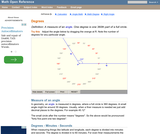Conditions of Use:
Read the Fine Print
Rating

An interactive applet that acts as a 'digital manipulative' for explaining angles measured in degrees. The applet has an angle formed from two segment that can be dragged around in a circle. The angle measure is shown against a 'clock face' calibrated in degrees. The measures can be turned off for class angle estimation discussions. Applet can be enlarged to full screen size for use with a classroom projector. This resource is a component of the Math Open Reference Interactive Geometry textbook project at http://www.mathopenref.com.

Subject:
Geometry
Material Type:
Reading
Simulation
Provider:
Math Open Reference
Author:
John Page
Date Added:
02/16/2011Conditions of Use:
Read the Fine Print
Rating

An interactive applet and associated web page that demonstrate the apothem of a regular polygon. The applet shows a regular polygon where the user can alter the number of sides and resize it by dragging any vertex. At all times, the apothem (line from center to a midpoint of a side) is drawn and it's length shown. The text on the page shows two formulae for calculating the apothem, depending on what you are give to start. Applet can be enlarged to full screen size for use with a classroom projector. This resource is a component of the Math Open Reference Interactive Geometry textbook project at http://www.mathopenref.com.

Subject:
Geometry
Material Type:
Reading
Simulation
Provider:
Math Open Reference
Author:
John Page
Date Added:
02/16/2011